TensorFlow 2.0 RC is available

# TensorBoard 直方图信息中心

TensorBoard 直方图信息中心用于显示在 TensorFlow 图中某些 Tensor 随着时间推移而变化的分布。即，该信息中心可显示在不同时间点对应张量的许多张直方图图示。

## 基本示例

import tensorflow as tf

k = tf.placeholder(tf.float32)

# Make a normal distribution, with a shifting mean
mean_moving_normal = tf.random_normal(shape=, mean=(5*k), stddev=1)
# Record that distribution into a histogram summary
tf.summary.histogram("normal/moving_mean", mean_moving_normal)

# Setup a session and summary writer
sess = tf.Session()
writer = tf.summary.FileWriter("/tmp/histogram_example")

summaries = tf.summary.merge_all()

# Setup a loop and write the summaries to disk
N = 400
for step in range(N):
k_val = step/float(N)
summ = sess.run(summaries, feed_dict={k: k_val})


tensorboard --logdir=/tmp/histogram_example


TensorBoard 运行时，在 Chrome 或 Firefox 加载该数据，并导航到直方图信息中心。然后我们可以即可看到正态分布数据的直方图图示。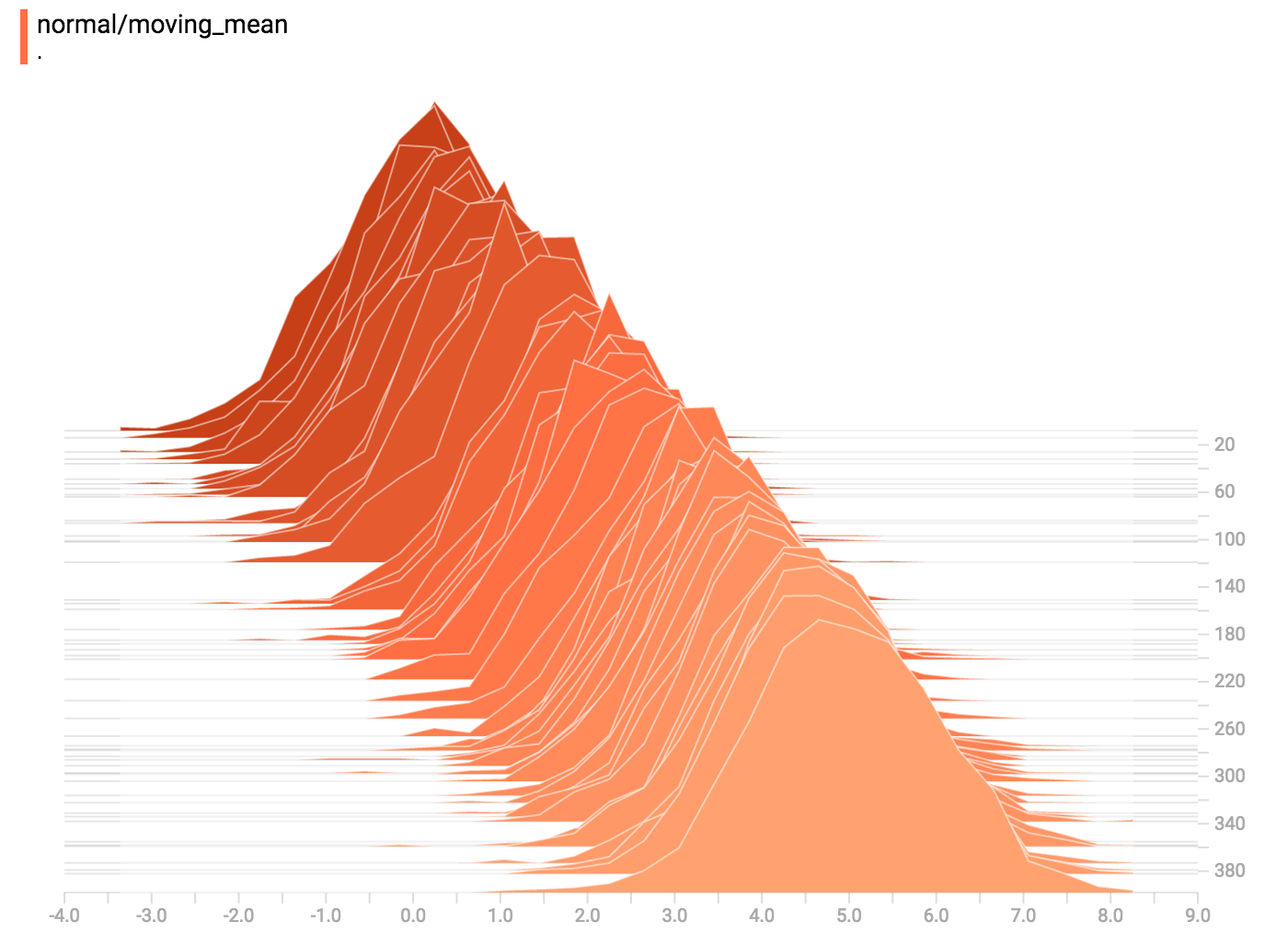tf.summary.histogram 接受任意大小和形状的张量，并将该张量压缩成一个由许多分箱组成的直方图数据结构，这些分箱有各种宽度和计数。例如，假设我们要将数字 [0.5, 1.1, 1.3, 2.2, 2.9, 2.99] 整理到不同的分箱中，我们可以创建三个分箱： * 一个分箱包含 0 到 1 之间的所有数字（会包含一个元素：0.5）， * 一个分箱包含 1 到 2 之间的所有数字（会包含两个元素：1.1 和 1.3）， * 一个分箱包含 2 到 3 之间的所有数字（会包含三个元素：2.2、2.9 和 2.99）。

TensorFlow 使用类似的方法创建分箱，但与我们的示例不同，它不创建整数分箱。对于大型稀疏数据集，可能会导致数千个分箱。相反，这些分箱呈指数分布，许多分箱接近 0，有较少的分箱的数值较大。 然而，将指数分布的分箱可视化是非常艰难的。如果将高度用于为计数编码，那么即使元素数量相同，较宽的分箱所占的空间也越大。反过来推理，如果用面积为计数编码，则使高度无法比较。因此，直方图会将数据重新采样并分配到统一的分箱。很不幸，在某些情况下，这可能会造成假象。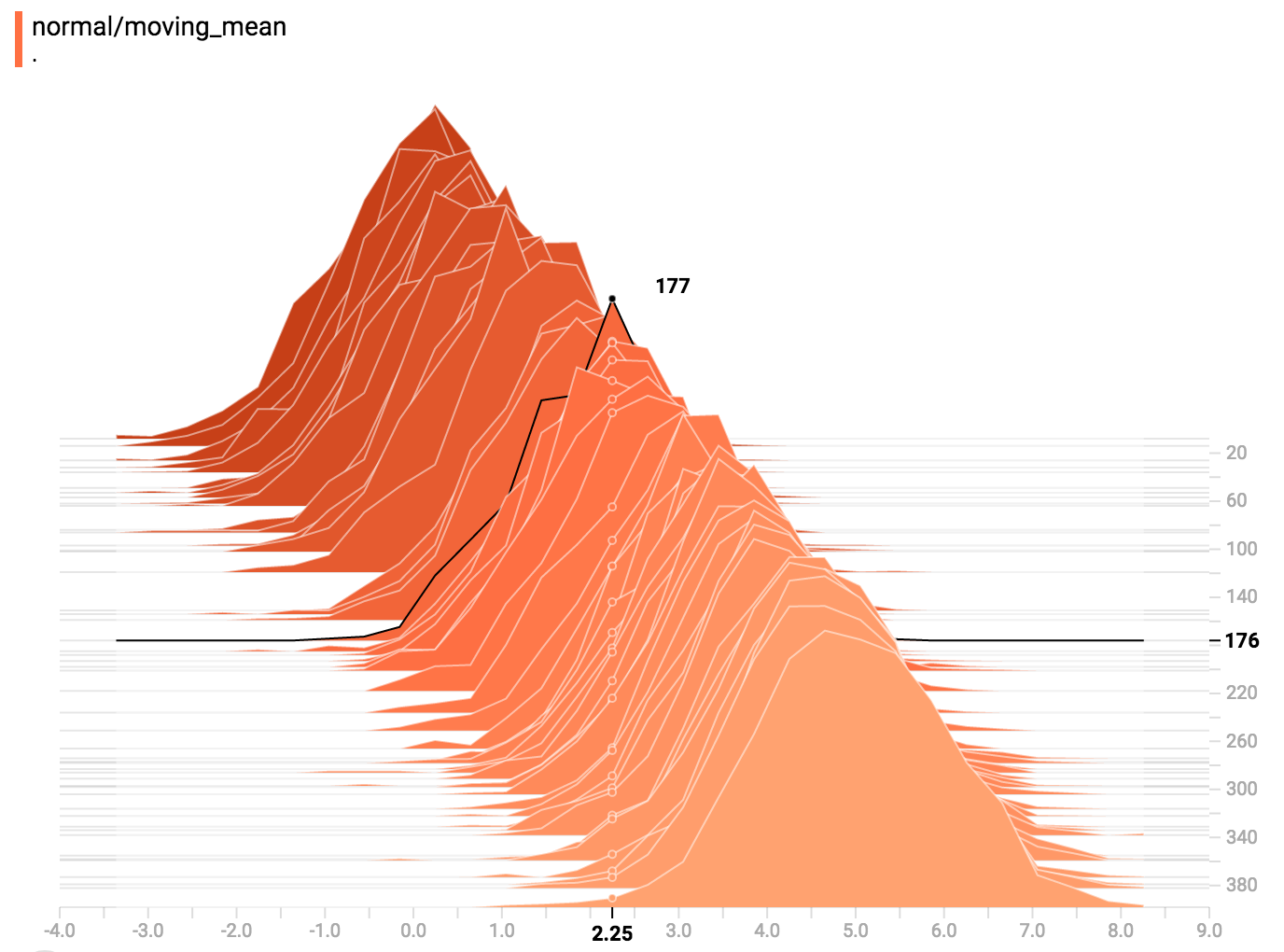## 覆盖模式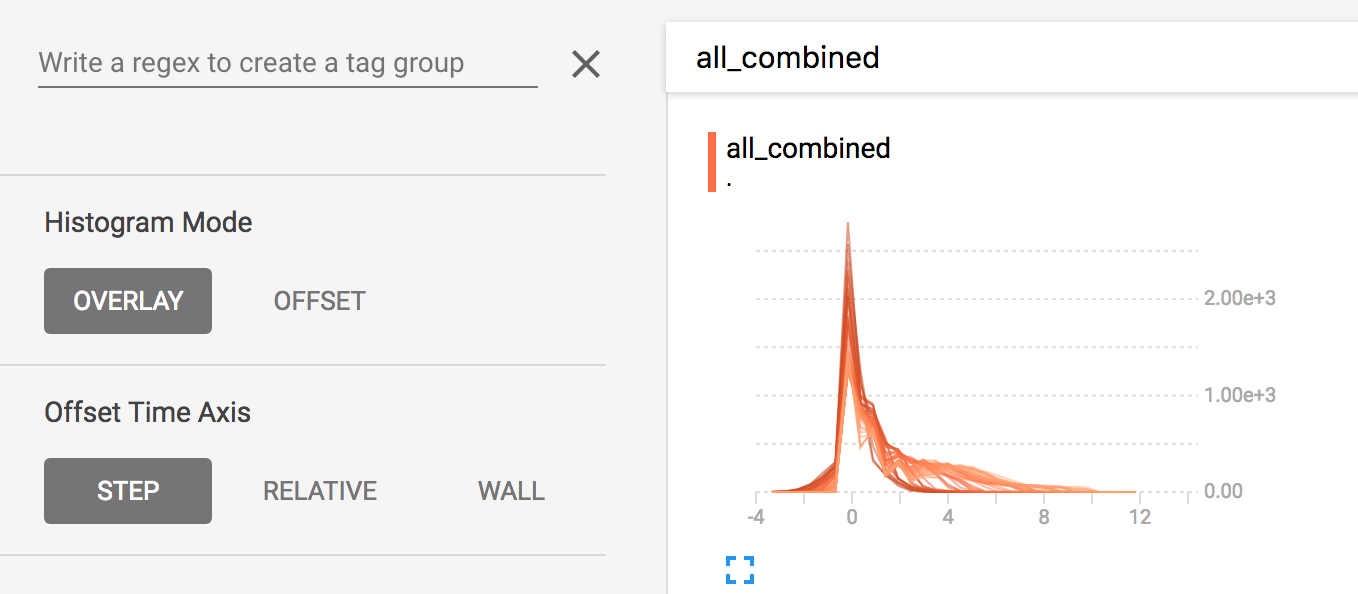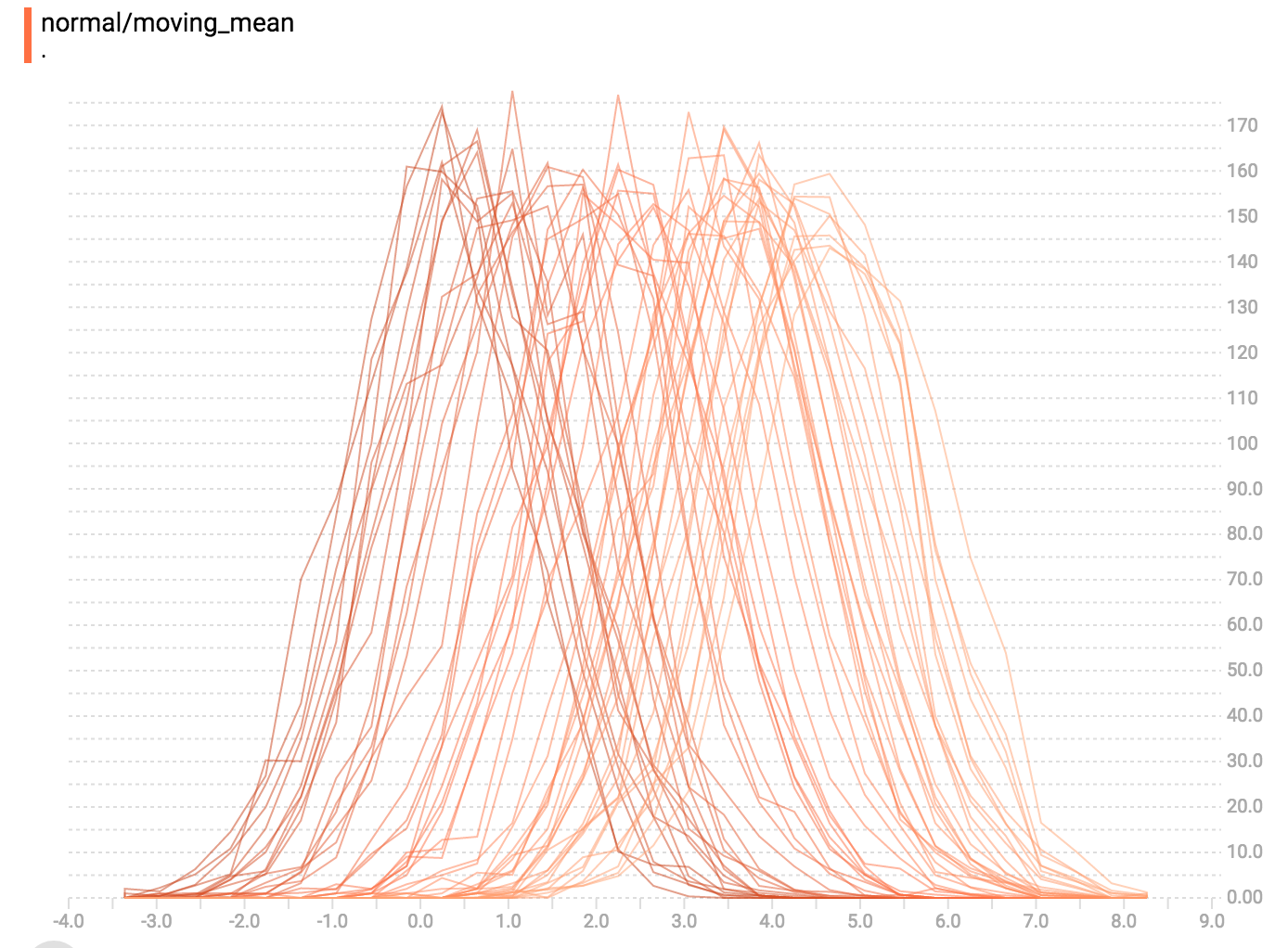现在，每个切片都是图表上的一条单独线条，y 轴显示的是每个分箱内的项目数。颜色较深的线条表示较早的步，而颜色较浅的线条表示较晚的步。同样，您可以将鼠标悬停在图表上以查看其他一些信息。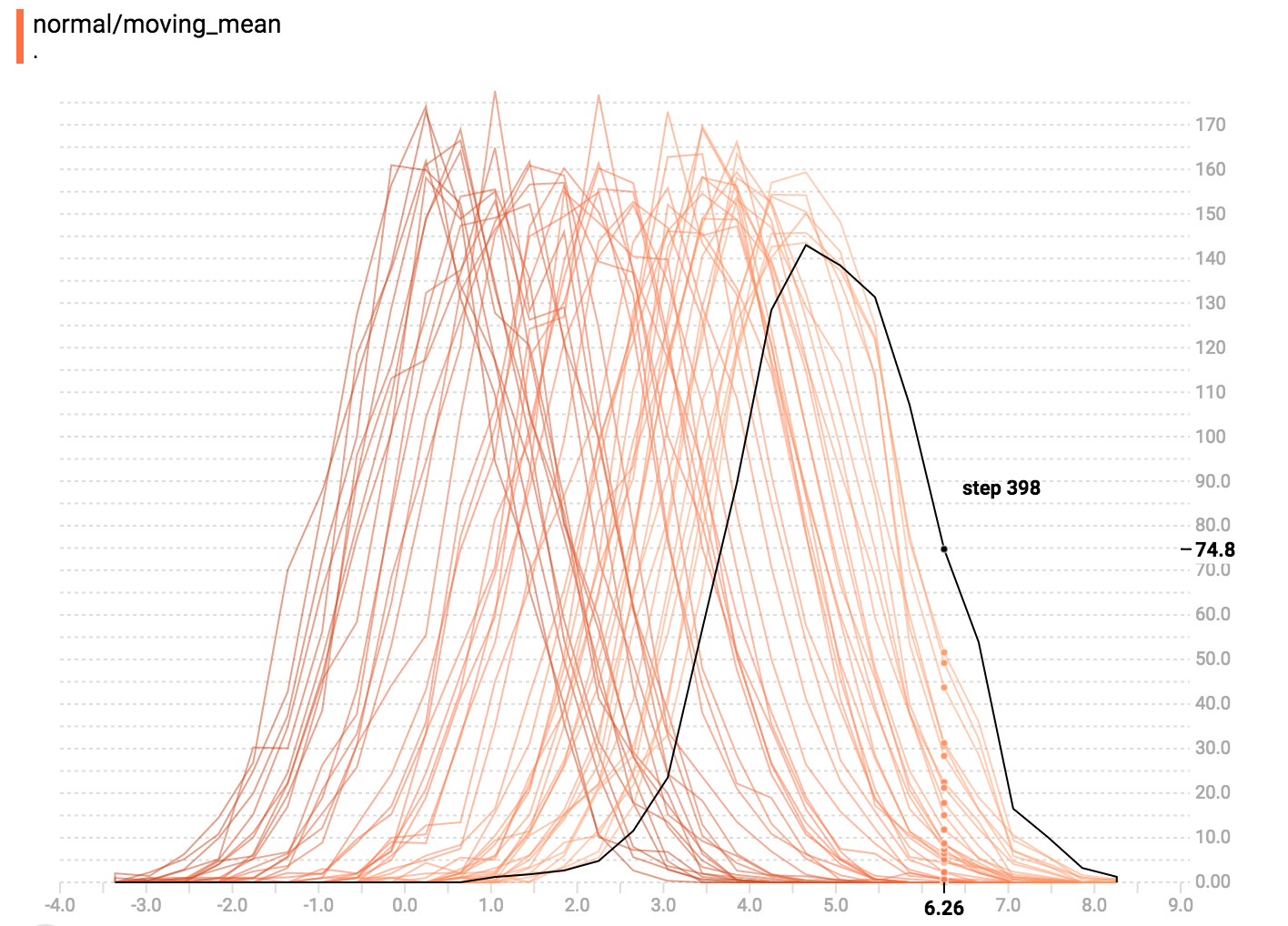## 多峰分布

import tensorflow as tf

k = tf.placeholder(tf.float32)

# Make a normal distribution, with a shifting mean
mean_moving_normal = tf.random_normal(shape=, mean=(5*k), stddev=1)
# Record that distribution into a histogram summary
tf.summary.histogram("normal/moving_mean", mean_moving_normal)

# Make a normal distribution with shrinking variance
variance_shrinking_normal = tf.random_normal(shape=, mean=0, stddev=1-(k))
# Record that distribution too
tf.summary.histogram("normal/shrinking_variance", variance_shrinking_normal)

# Let's combine both of those distributions into one dataset
normal_combined = tf.concat([mean_moving_normal, variance_shrinking_normal], 0)
# We add another histogram summary to record the combined distribution
tf.summary.histogram("normal/bimodal", normal_combined)

summaries = tf.summary.merge_all()

# Setup a session and summary writer
sess = tf.Session()
writer = tf.summary.FileWriter("/tmp/histogram_example")

# Setup a loop and write the summaries to disk
N = 400
for step in range(N):
k_val = step/float(N)
summ = sess.run(summaries, feed_dict={k: k_val})


## 更多分布

import tensorflow as tf

k = tf.placeholder(tf.float32)

# Make a normal distribution, with a shifting mean
mean_moving_normal = tf.random_normal(shape=, mean=(5*k), stddev=1)
# Record that distribution into a histogram summary
tf.summary.histogram("normal/moving_mean", mean_moving_normal)

# Make a normal distribution with shrinking variance
variance_shrinking_normal = tf.random_normal(shape=, mean=0, stddev=1-(k))
# Record that distribution too
tf.summary.histogram("normal/shrinking_variance", variance_shrinking_normal)

# Let's combine both of those distributions into one dataset
normal_combined = tf.concat([mean_moving_normal, variance_shrinking_normal], 0)
# We add another histogram summary to record the combined distribution
tf.summary.histogram("normal/bimodal", normal_combined)

# Add a gamma distribution
gamma = tf.random_gamma(shape=, alpha=k)
tf.summary.histogram("gamma", gamma)

# And a poisson distribution
poisson = tf.random_poisson(shape=, lam=k)
tf.summary.histogram("poisson", poisson)

# And a uniform distribution
uniform = tf.random_uniform(shape=, maxval=k*10)
tf.summary.histogram("uniform", uniform)

# Finally, combine everything together!
all_distributions = [mean_moving_normal, variance_shrinking_normal,
gamma, poisson, uniform]
all_combined = tf.concat(all_distributions, 0)
tf.summary.histogram("all_combined", all_combined)

summaries = tf.summary.merge_all()

# Setup a session and summary writer
sess = tf.Session()
writer = tf.summary.FileWriter("/tmp/histogram_example")

# Setup a loop and write the summaries to disk
N = 400
for step in range(N):
k_val = step/float(N)
summ = sess.run(summaries, feed_dict={k: k_val})


### 伽玛分布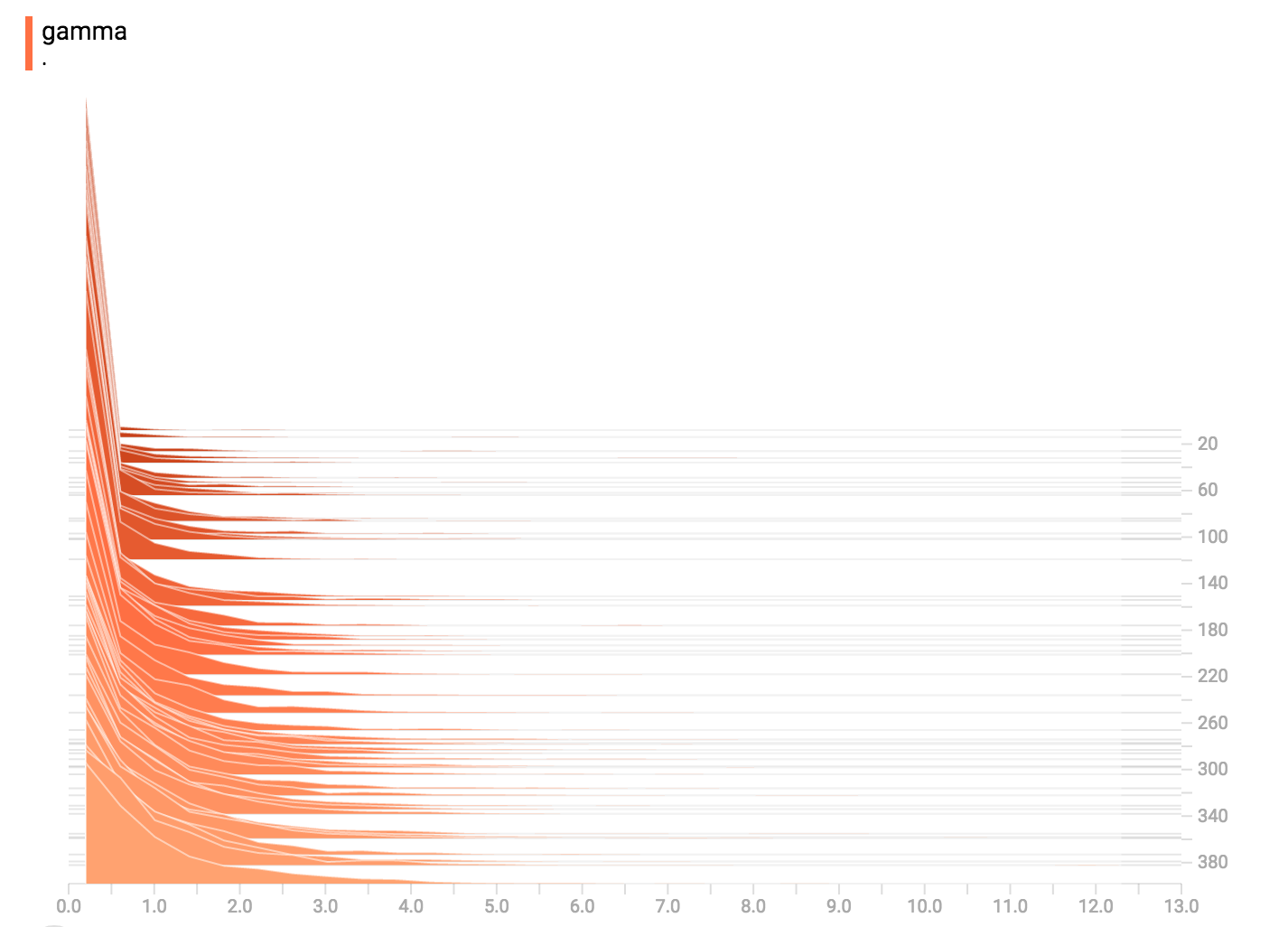### 均匀分布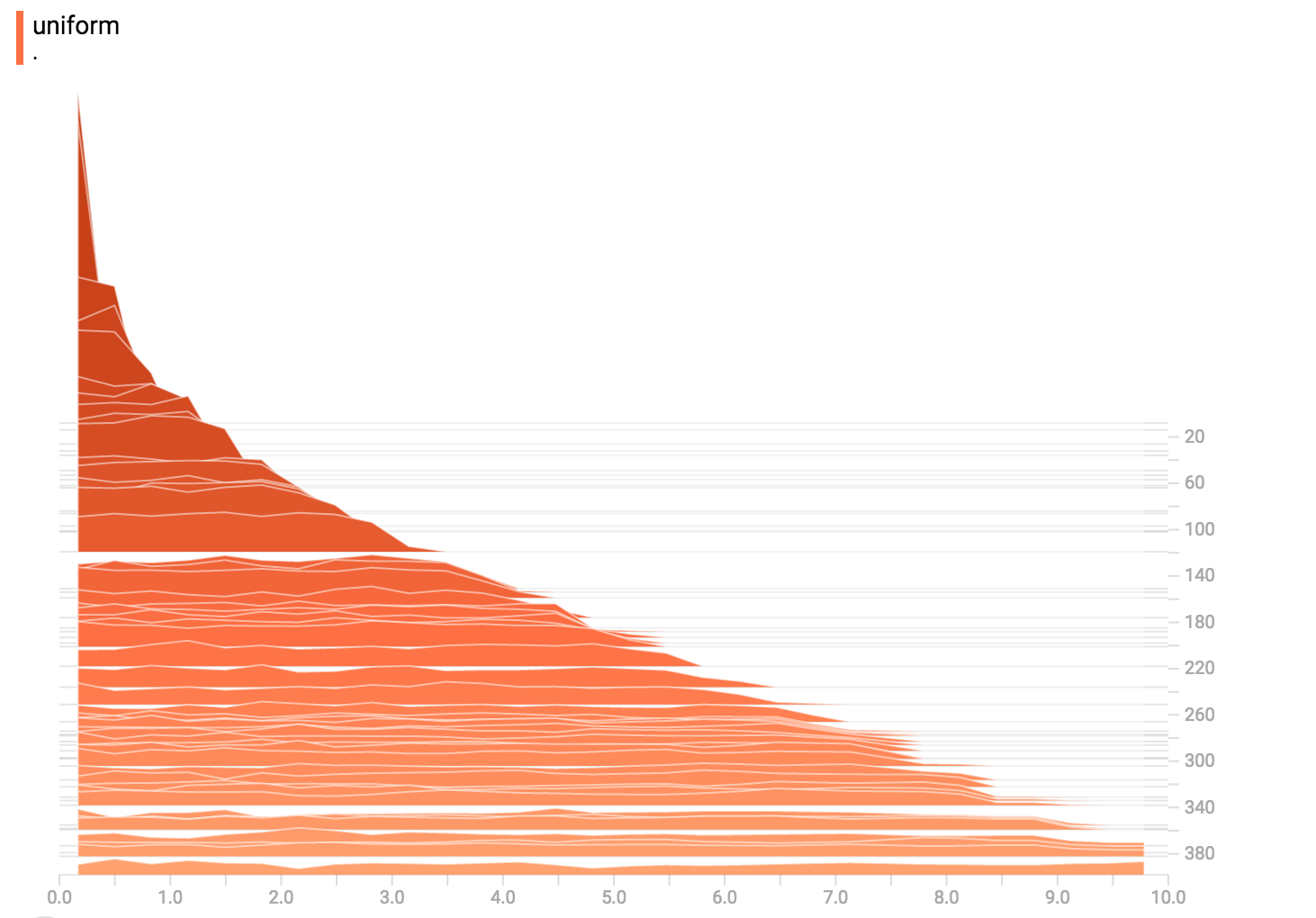### 泊松分布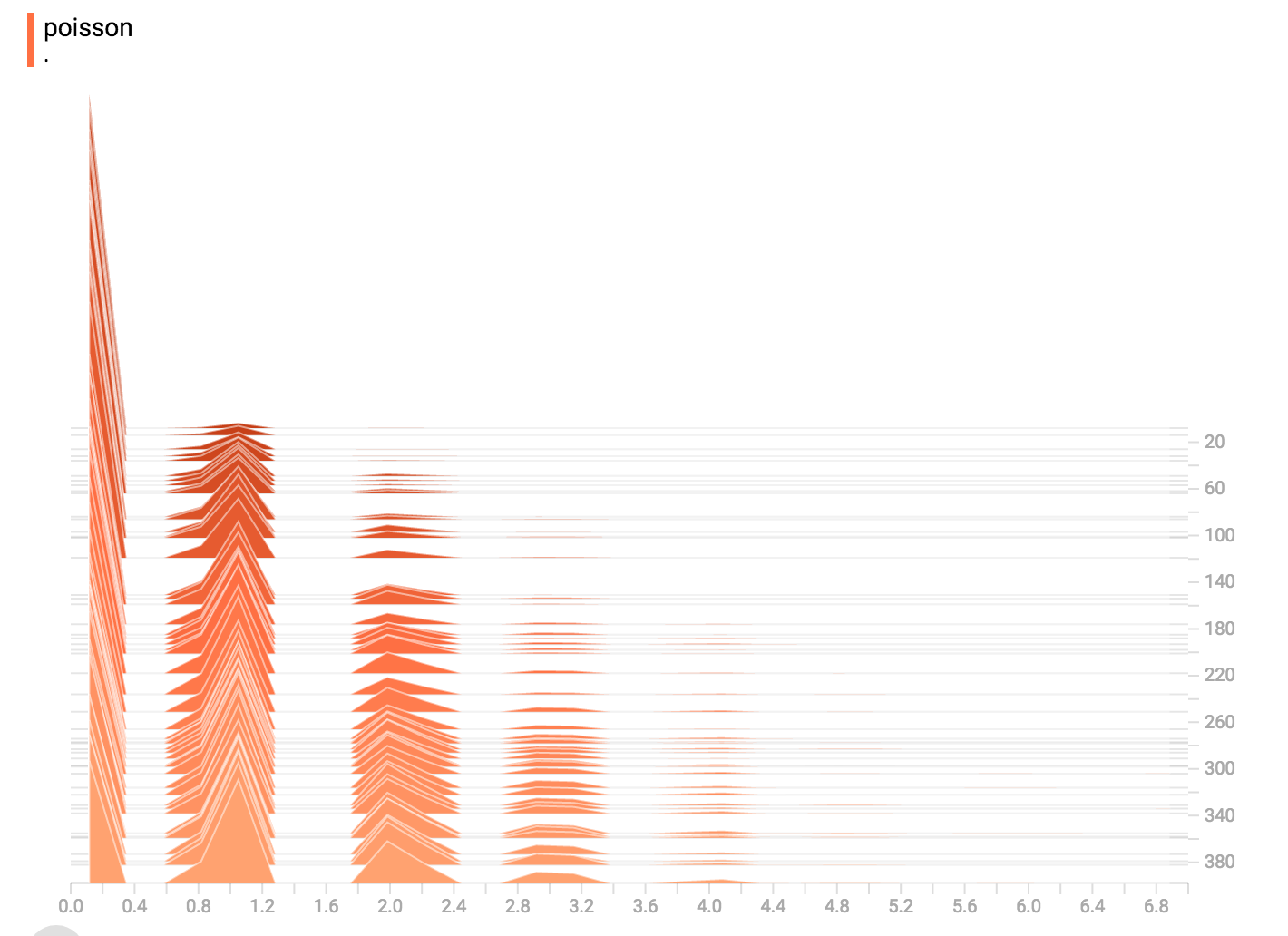泊松分布以整数为基础。所以，所有生成的值都完全是整数。直方图压缩将数据移动到按浮点数划分的分箱中，导致生成的图表在整数值上显示出很小的隆起，而不是锐利的尖峰。